1. A field line is normal to an equipotential surface
2. The mass of a planet is M and its radius is R. In order for a body of mass m to escape the gravitational attraction of the planet, its kinetic energy at the surface of the planet must be at least
3. The escape speed of a rocket from the surface of Earth depends on the universal gravitational constant G. Other factors that may affect the escape speed are the

I. mass of Earth
II. radius of Earth
III. mass of the rocket.

Which of the above factors is/are correct?

4. Kindly refer the question below:

What is the unit of 0, where G is the gravitational constant and ε0 is the permittivity of free space?

5. In an experiment, oil droplets of mass m and charge q are dropped into the region between two horizontal parallel plates. The electric field E between the plates can be adjusted. Air resistance is negligible. Which is correct when the droplets fall vertically at constant velocity?
6. A positive point charge is placed above a metal plate at zero electric potential. Which diagram shows the pattern of electric field lines between the charge and the plate?
7. A satellite at the surface of the Earth has a weight W and gravitational potential energy Ep. The satellite is then placed in a circular orbit with a radius twice that of the Earth.

What is the weight of the satellite and the gravitational potential energy of the satellite when placed in orbit ?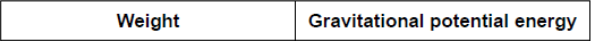8. The diagram shows two point charges P and Q. At which position is the electric field strength equal to zero?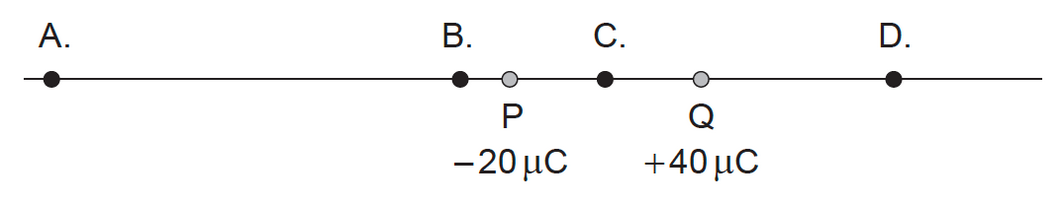9. Two spherical objects of mass M are held a small distance apart. The radius of each object is r.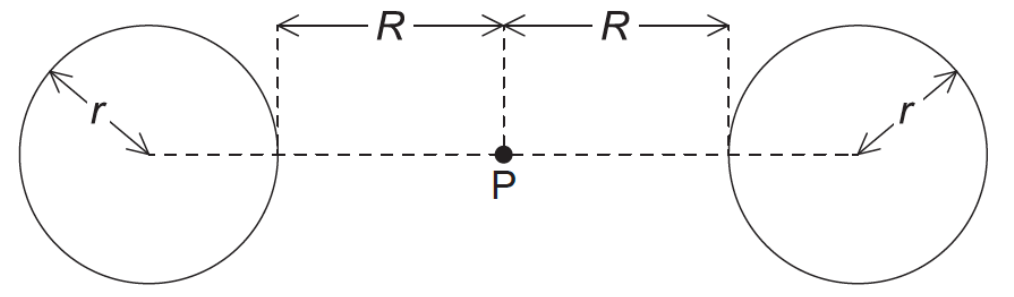Point P is the midpoint between the objects and is a distance R from the surface of each object. What is the gravitational potential at point P?

10. The diagram shows equipotential lines due to two objects.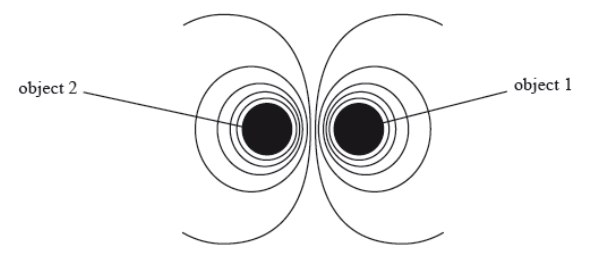The two objects could be

11. The diagram shows 5 gravitational equipotential lines. The gravitational potential on each line is indicated.

A point mass m is placed on the middle line and is then released. Values given in MJ kg–1.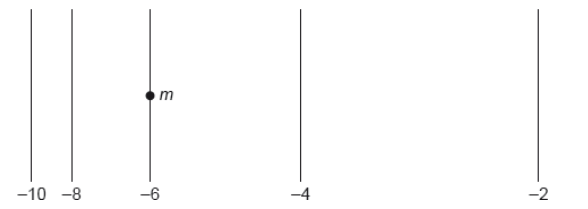Which is correct about the direction of motion and the acceleration of the point mass?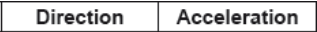12. At the surface of a planet of radius r, the gravitational field strength is g and the gravitational potential is V. Which gives the gravitational field strength and gravitational potential at a height 3r above the surface?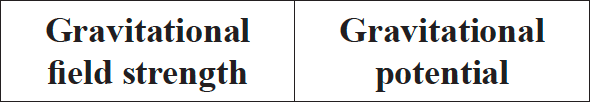13. A positive point charge P and a negative point charge Q of equal magnitude are held at fixed positions. Y is a point midway between P and Q.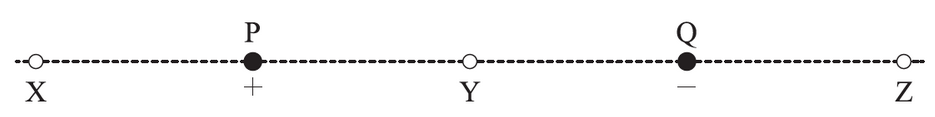Which of the following gives the direction of the electric field due to the charges at X, Y and Z?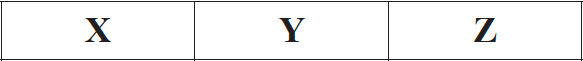14. A satellite is moved from a low orbit to a higher orbit. Which of the following accurately describes the energy of the satellite?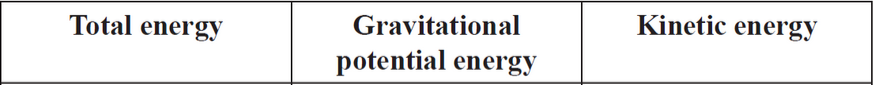15. Kindly refer the question below:

The sketch graph shows how the gravitational potential V of a planet varies with distance r from the centre of the planet of radius R0.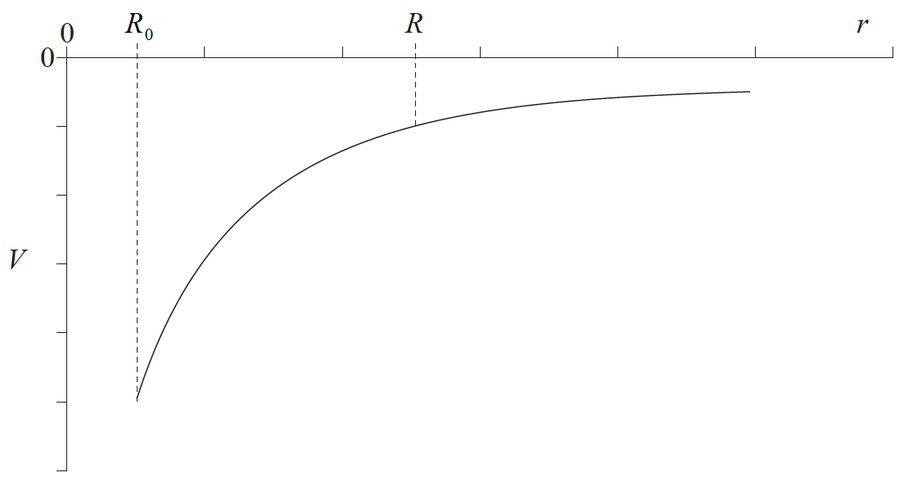The magnitude of the gravitational field strength at the point r = R equals the

16. A spacecraft moves towards the Earth under the influence of the gravitational field of the Earth.

The three quantities that depend on the distance r of the spacecraft from the centre of the Earth are the

I.   gravitational potential energy of the spacecraft
II   gravitational field strength acting on the spacecraft
III. gravitational force acting on the spacecraft.

Which of the quantities are proportional to 1/r^2?

17. Kindly refer the question below:

An electron of mass me orbits an alpha particle of mass mα in a circular orbit of radius r. Which expression gives the speed of the electron?

18. Two positive and two negative point charges of equal magnitude are placed at the vertices of a square as shown. The origin of the axes is at the centre of the square.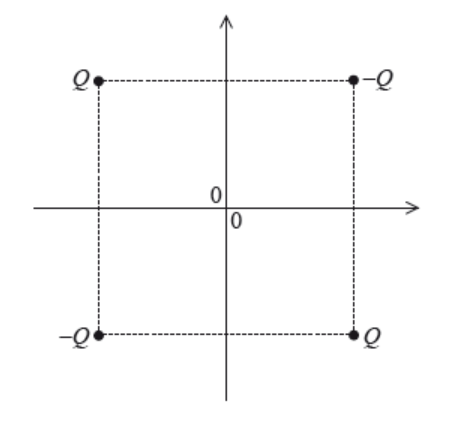The electric potential is zero

19. A moon of mass M orbits a planet of mass 100M. The radius of the planet is R and the distance between the centres of the planet and moon is 22R.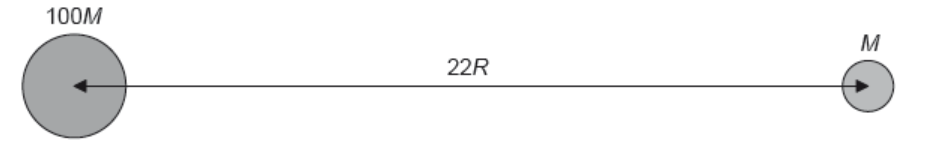What is the distance from the centre of the planet at which the total gravitational potential has a maximum value?

20. Kindly refer the question below:

The mass of the Earth is ME and the mass of the Moon is MM. Their respective radii are RE and RM.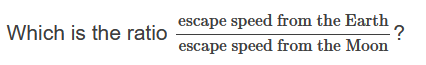21. Kindly refer the question below:

The diagram below shows a particle with positive charge q accelerating between two conducting plates at potentials and .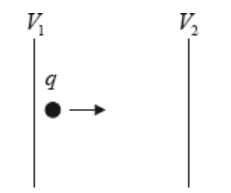Which of the following is the kinetic energy gained by the charge in moving between the plates?

22. A negatively charged particle falls vertically into a region where there is an electric field. The equipotentials of this field are shown.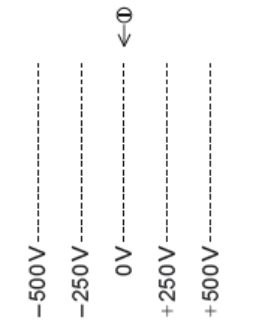What is the path followed by the particle?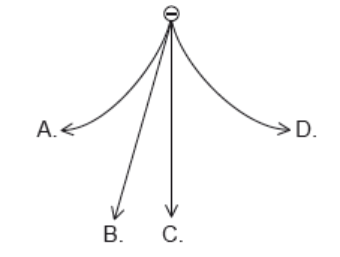23. Which of the following experiments provides evidence for the existence of matter waves?
24. The two graphs below represent the variation with distance, d, for d = r to d = 2r of the electric field and the electric potential around an isolated point charge.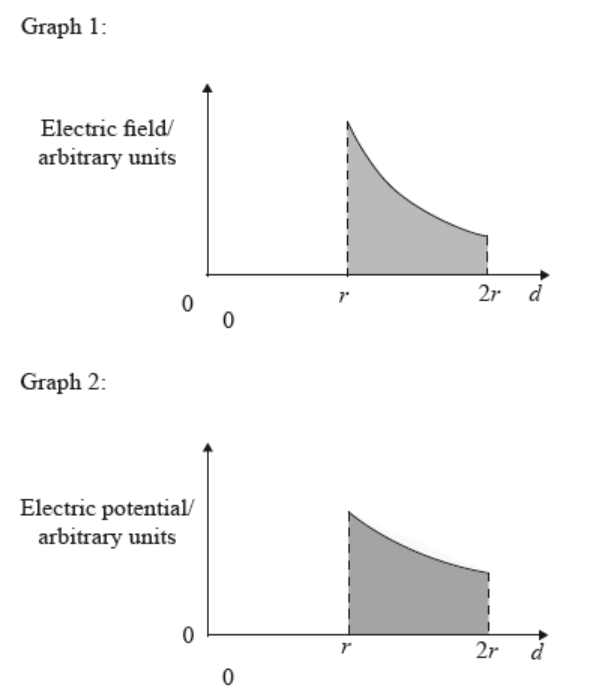The work done by an external force in moving a test charge from to is equal to multiplied by the

25. A charge of −3 C is moved from A to B and then back to A. The electric potential at A is +10 V and the electric potential at B is −20 V. What is the work done in moving the charge from A to B and the total work done?26. Which of the following represents a scalar and a vector quantity?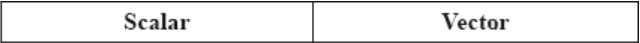27. Gravitational potential at a point is defined as the work done
28. Four identical, positive, point charges of magnitude Q are placed at the vertices of a square of side 2d. What is the electric potential produced at the centre of the square by the four charges?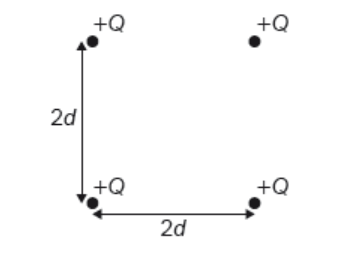29. The diagram shows two parallel metal plates X and Y.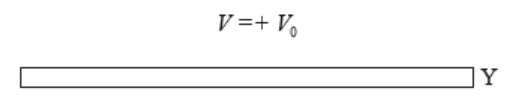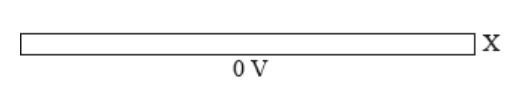Plate X is at Earth potential (0 V) and the potential of plate Y is .

Which of the following is correct in respect of the magnitude and the direction of the electric field between the plates?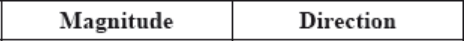30. The escape speed from the surface of a planet depends on
31. M is a spherical mass situated far away from any other masses. Which of the following represents gravitational equipotential surfaces having constant potential difference between them?